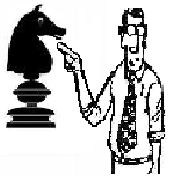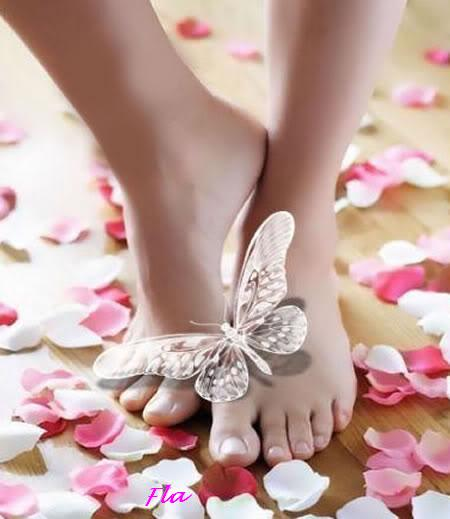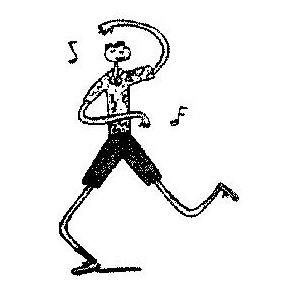# Barney & Clyde by Gene Weingarten, Dan Weingarten & David Clark for November 12, 2013

1.##### yousirover 7 years ago

Googol it.

2.##### black_knight15_auover 7 years ago

Hint both the volume and surface area of the top are ….

3.##### QuietStorm27over 7 years ago

Me too.

4.##### Riff GibsonPremium Memberover 7 years ago

Solution for all our non-math-geek “Barney & Clyde” friends:

Since the end (base) of a cylinder [=the cake] is a circle, the area of that circle [=top of the cake] is given by “pi” multiplied by the radius squared [= “pi” x “r” x “r”].

Multiplying by the height of the cake we get the volume of the cylinder, er, cake: “height” multiplied by “pi” multiplied by “radius squared” [= “h” x “pi” x “r” x “r”]

So, if both the radius and the height are one unit, then the above multiplication yields both the area and the volume equal to pi:

Area of the top of the cake = “pi” x “r” x “r” = “pi” x 1 × 1 = “pi”

Volume of the cake = “h” x “pi” x “r” x “r” = 1 x “pi” x 1 × 1 = “pi”

Therefore, in this case, the cake is also pi!